# What is the Transformer Capacity? How to Calculate and Test it?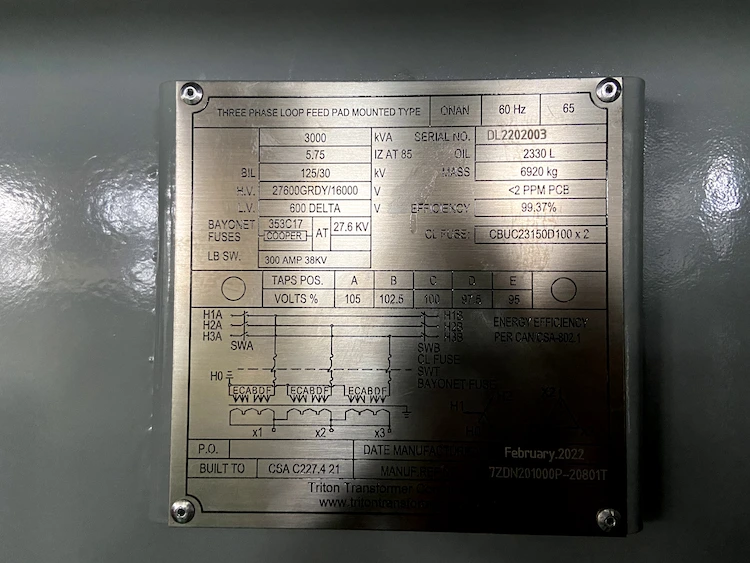The rated transformer capacity is the apparent power value input to the transformer, including the active power and reactive power absorbed by the transformer itself.

Methods for judging transformer capacity include:

1) Measure the DC resistance of the transformer to determine the capacity of the transformer;
2) Measurement with a capacity tester is carried out through a low short-circuit current test using a transformer capacity tester.

In working practice, it is necessary to determine the actual capacity of the transformer without the nameplate or the nameplate does not match.

There are many kinds of measurement methods, most of which require complex tests and calculations, and are not easily operable on site.

This article will introduce two practical methods of transformer capacity testing that are currently available.

## What is the rated capacity of a three-phase transformer?

The formula for calculating the rated capacity of a three-phase transformer is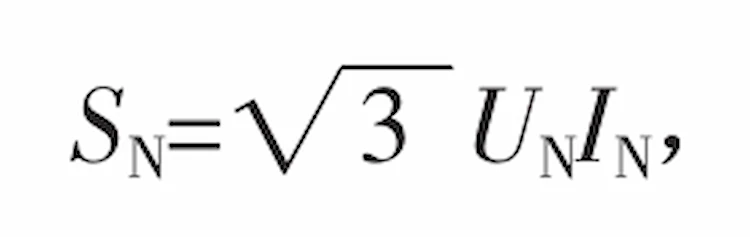In the formula, SN is the rated capacity of the transformer, and the unit is kVA;

UN is the rated voltage of the transformer, the unit is kV, the step-down transformer can be selected from 6, 10, 20, 35, 66, 110, 220, 330, 500 kV;

IN is the rated current of the transformer, in A.

It can be seen from formula (1) that to calculate SN, only the data of IN can be obtained. UN is a known value, and since UN is connected to the power system, it is not possible for the transformer to deviate widely from the system voltage.

From the definition of transformer short-circuit impedance, it can be known that the calculation formula of short-circuit impedance Uk is: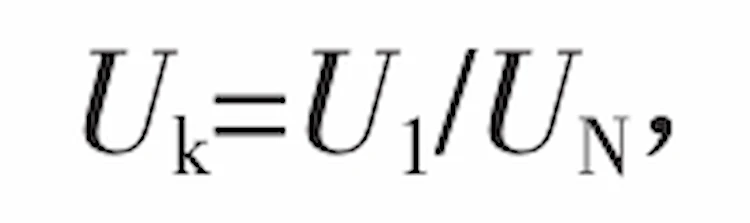In the formula, Uk is the short-circuit impedance, and the common oil-immersed transformer of 1600 kVA and below is 4% or 4.5%;

U1 is the voltage value of the primary side when the voltage is applied to the primary side of the transformer, the secondary side is short-circuited and its short-circuit current reaches the rated value;

UN is the rated voltage value of the primary side of the transformer.

## What is the principle of transformer current test?

The principle of short-circuit test is analyzed below. The principle of rated short-circuit current test is shown in Figure 1.

In Figure 1, I1N and I2N are the primary and secondary rated currents of the rated short-circuit test, respectively.

Assuming that the transformer under test is 1000 kVA, I1N and I2N can reach 57.7 and 1448 A, respectively.

It can be seen that under the rated conditions, the current and power supply capacity of the short-circuit test are large.

(1)When using rated current for short-circuit test, the test power supply capacity Sk should be determined according to formula (3), that is,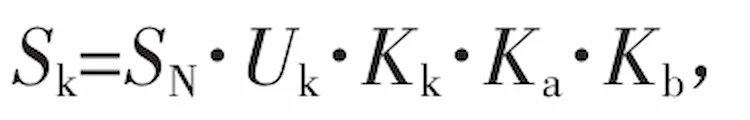In the formula, Uk is the impedance voltage percentage, and it is 4.5% for a 1000 kVA oil-immersed transformer;

Kk is the test current capacity factor, 0.062 5~1.0, generally 1.0 as far as possible;

Ka is the transformation factor, generally 1.2~1.3;

Kb is a safety factor, preferably 1.0~1.1;

SN is the rated capacity of the tested transformer.

It can be seen from formula (3) that for a 1000 kVA transformer, the required power supply capacity during short-circuit test

Sk=1 000×4.5%×1.0×1.2×1.1=59.4kVA.

The calculated test capacity is very large, and it is difficult to obtain this condition in the user test site.

(2)When the voltage and current of the transformer are within the rated range for short-circuit test, the voltage and current have a linear proportional relationship, that is, the current increases with the increase of the primary side test voltage, and a low short-circuit current can be used for the short-circuit test, as shown in the figure 2 shown. So getIn the formula, U1′ is the primary side voltage value of the low short-circuit current test, and I1′ is the primary side current value of the low short-circuit current test.

Formula (4) can be deduced that the primary side current value of the rated short-circuit current test is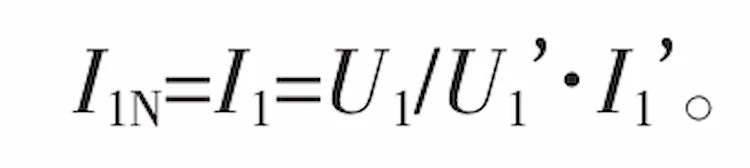From equations (1) and (5), the formula for rated capacity can be deduced asFrom equations (2) and (6), it can be deduced that the required transformer capacity calculation formula is as followsExample Now an oil-immersed transformer is tested with low short-circuit current, its Uk=4.5%, UN=10 kV.

The measured data are: U1’=5.63 V (power factor has been considered), I1’=0.45 A, then the capacity SN= 3 × 10 000 × 10 000 × 4.5%/5.63 × 0.45=622 966 VA. The nominal rated capacity corresponding to this transformer should be 630 kVA.

## How to calculate short circuit impedance of transformer?

According to formula (7), because the impedance characteristic of the tested transformer Uk is fixed, and the voltage U1′ applied during the test is small, the value of I1’/U1’=K1 is stable, and UN is a known number.

Therefore, it is concluded that SN is proportional to Uk, that is,Therefore, it can be deduced that the short-circuit impedance of the transformer is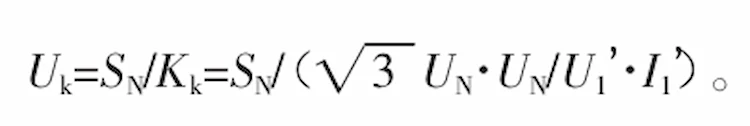## How to determine the transformer capacity?

The measurement of the DC resistance of the transformer winding is an essential transformer test item.

Through the measurement of DC resistance, it is not only possible to know whether there is a phase-to-phase or inter-turn short circuit inside the transformer, but also to analyze the capacity of the transformer by comparison.

The larger the transformer capacity, the smaller the primary and secondary DC resistance values, which are inversely proportional.

When testing, it is only necessary to test the DC resistance value of the transformer to be tested according to the regulations, and compare the average value of the DC resistance value of the middle gear on the high-voltage side and the average value of the DC resistance value of the low-voltage side with the reference average value of the corresponding transformer, that is, The capacity range of the transformer can be analyzed.

This method is simple and convenient to operate on site, but it also has certain limitations.

Because there are many manufacturers of transformers, there are many types of transformer specifications, the same capacity, different manufacturers, and different specifications and models of transformers have a certain gap in resistance value. There is no strict limit on the resistance value of transformers in China’s national standard for transformers. The resistance value of the transformer of the manufacturer and model is relatively difficult.

Therefore, judging the rated capacity of the transformer by the simple method of judging the capacity of the transformer by the DC resistance value can only make a preliminary judgment on the rated capacity of the conventional distribution transformer.

## Common problems in transformer capacity testing

At present, it is recommended to use the principle of low short-circuit current with the transformer capacity test device to test the transformer capacity.

Sometimes, the problem of falsification of the transformer nameplate data is encountered, mainly including the falsification of the rated capacity of the transformer and the simultaneous falsification of the rated capacity of the transformer and the impedance voltage.

(1) For those only the rated capacity of the transformer is false, the measured capacity of the transformer can be measured by directly inputting the data including the impedance voltage with the capacity tester.

(2) If the transformer and the rated capacity are false together, the impedance voltage should be further analyzed.

To analyze the impedance voltage, the data including the rated capacity and impedance voltage on the transformer nameplate can be input into the capacity tester to measure whether the impedance voltage of the transformer is normal.

According to the relationship between the transformer impedance voltage and the rated capacity, if the measured impedance voltage and the corresponding transformer national standard impedance voltage have a deviation of 10~15%, it can be inferred that there is a problem with the rated capacity of the transformer and the impedance voltage.

At this time, the impedance voltage of the national standard is used to test the capacity of the transformer.

The impedance voltage on the nameplate is not used.

When analyzing the capacity, it is impossible to determine whether the nominal impedance voltage on the nameplate is true. Generally, a capacity test device should be used to measure the load loss of the transformer and the impedance voltage value for comparison.

## How to test the capacity of large-capacity special transformers?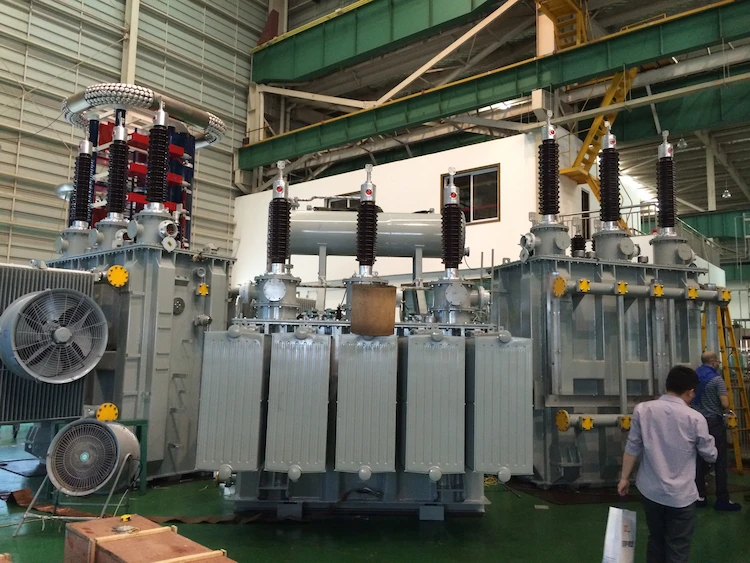For large-capacity special transformers, the difficulty of capacity testing is mainly the judgment of impedance voltage value.

In the case of voltage value, there is also a big problem in using the nominal impedance voltage value on the transformer nameplate as the test value:

(1) The nominal impedance voltage value on the transformer nameplate may be a false value;

(2) There is also a great risk when judging the nominal impedance voltage value on the transformer nameplate by experience.

Therefore, for the test of large-capacity special transformers, according to the actual situation, you can first use a capacity tester to test the impedance voltage value of the transformer, and then use the tested impedance voltage value as one of the input data, and use the transformer capacity tester to test the capacity of the special transformer. the size of.

## Summarize:

The capacity of conventional small-capacity transformers can be judged by a simple method of judging the capacity of transformers with empirical resistance values.

For more scientific judgment, it is recommended to use a distribution transformer capacity test device based on short-circuit impedance to test.

When encountering special circumstances, such as the capacity test of large-capacity or special transformers, in addition to using the existing capacity tester, it is necessary to use various information to assist judgment, including the size of the transformer, and whether there is any witness to support the false information of the transformer. etc. to make a comprehensive judgment. When conditions permit, the participation and support of transformer manufacturers are also required.

### 10 kv Oil Filled Transformer

Fault Diagnosis and Analysis of 10 kv Oil Filled Transformer 10 kv Oil filled transformer